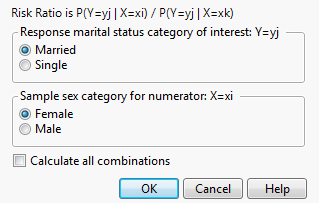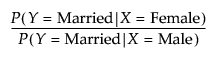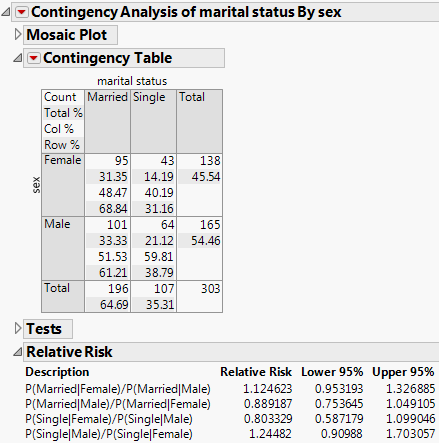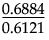Publication date: 11/29/2021

## Example of the Relative Risk Option

This example examines the relative probabilities of being married and single for the participants in a poll.

1. Select Help > Sample Data Library and open Car Poll.jmp.

2. Select Analyze > Fit Y by X.

3. Select marital status and click Y, Response.

4. Select sex and click X, Factor.

5. Click OK.

6. Click the red triangle next to Contingency Analysis of marital status By sex and select Relative Risk.

The Choose Relative Risk Categories window appears.

Figure 7.14 The Choose Relative Risk Categories WindowNote the following about the Choose Relative Risk Categories window:

If you are interested in only a single response and factor combination, you can select that here. For example, if you clicked OK in the window in Figure 7.14, this is the calculation:If you would like to calculate the risk ratios for all (2 × 2 = 4) combinations of response and factor levels, select the Calculate All Combinations check box (Figure 7.15).

7. Ask for all combinations by selecting the Calculate All Combinations check box. Leave all other default selections as is.

Figure 7.15 Example of the Risk Ratio ReportThe relative risk is calculated as follows:

1. Examine the first entry in the Relative Risk report, which is P(Married|Female)/P(Married|Male).

2. You can find these probabilities in the Contingency Table. Since the probabilities are computed based on two levels of sex, which differs across the rows of the table, use the Row% to read the probabilities:

P(Married|Female) = 0.6884

P(Married|Male) = 0.6121

Therefore, the relative risk is calculated as follows:

P(Married|Female)/P(Married|Male) == 1.1247